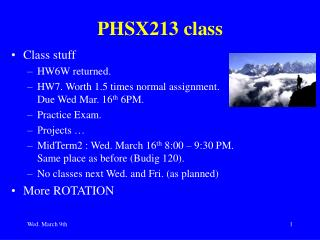DownloadDownload PresentationPHSX213 class

PHSX213 class

Download PresentationPHSX213 class

- - - - - - - - - - - - - - - - - - - - - - - - - - - E N D - - - - - - - - - - - - - - - - - - - - - - - - - - -
Presentation Transcript

1. PHSX213 class • Class stuff • HW6W returned. • HW7. Worth 1.5 times normal assignment. Due Wed Mar. 16th 6PM. • Practice Exam. • Projects … • MidTerm2 : Wed. March 16th 8:00 – 9:30 PM. Same place as before (Budig 120). • No classes next Wed. and Fri. (as planned) • More ROTATION

2. Rotational Inertia Demo

3. Work and Rotational KE • We saw for linear motion that, W = ∫ F dx = DK = Kf – Ki = ½ m (vf2 – vi2) For rotational motion about a fixed axis, W = ∫ t dq = DK = Kf – Ki = ½ I (wf2 – wi2)

4. Example 10.78 • Pulley, uniform disk, mass M=0.5 kg, radius R=0.12m. • Mass m1=0.4 kg, m2 = 0.6 kg • Cord doesn’t slip. Disk rotates freely (ignore friction). • What is the magnitude of the acceleration of the blocks? • What is the tension in each cord ?

5. Reading Quiz • Which force gives the torque needed for objects to roll without slipping: • A) normal force • B) force due to gravity • C) kinetic friction • D) static friction do rolling example demo in parallel

6. Reading Quiz • Which force gives the torque needed for objects to roll without slipping: • A) normal force • B) force due to gravity • C) kinetic friction • D) static friction

7. Check-Point 1 • A wheel rolls without slipping along a horizontal surface. The center of the disk has a translational speed, v. The uppermost point on the wheel has a translational speed of : • A) 0 • B) v • C) 2v • D) need more information

8. Rolling A rolling object can be considered to be rotating about an axis through the CoM while the CoM moves.

9. Rolling • The rotational motion obeys St = ICoMawhile the translational motion obeys SF = m aCoM • Note that point P in contact with the ground has zero velocity ie. stationary. So static friction is what matters at that point. • Not convinced it is stationary – think about clean (unsmudged) tire tracks in the snow

10. Check-Point 2 A solid disk and a ring roll down an incline. The ring is slower than the disk if A. mring= mdisk, where m is the inertial mass. B. rring= rdisk, where r is the radius. C. mring= mdiskand rring= rdisk. D. The ring is always slower regardless of the relative values of m and r.

11. Forces in Rolling • Rolls smoothly down the ramp without sliding/slipping. • How much torque about the CoM does each force produce ? • How big is fS ? • How big is the acceleration ? Note : I’ll define +x down the incline

12. One can derive that : fS = I a/R2 = [b/(1+b)] mg sinq And that, a = g sinq/(1 + I/(MR2)) = g sinq/(1+b) Where we have defined b, via I = b M R2 bhoop = 1, bcylinder = 0.5, bsolid sphere = 0.4 Rolling

13. Discuss rolling in terms of KE • From energy conservation considerations. • Note the frictional force in this case doesn’t oppose the angular motion, and the work done by this non-conservative force is transformed into rotational kinetic energy. • W = ∫ t dq • And we find that K = ½ m v2 + ½ I w2 • = ½ m (1+b) v2 ( = mg h) Since the contact point doesn’t move, there is no translational displacement.

14. Another way to look at this • Stationary observer sees rotation about an axis at point P with w = v/R. • Using the parallel axis theorem, IP = ICoM + mR2 • So, K = ½ IPw2 • = ½ ICoMw2 +½ m v2 • just as before.

15. Rolling Body on Inclined Plane Demo

16. Loop-the-loop

17. Torque Definition for a Particle t = r F Vector product means that the torque is directed perpendicular to the plane formed by (r, F). Whether it is up or down is from the right-hand rule convention NB Only makes sense to talk about the torque wrt or about a certain point

18. Angular Momentum Demo

19. Angular Momentum Definition for a Particle l = r p = r m v NB Only makes sense to talk about the angular momentum wrt or about a certain point

20. Angular Momentum Definition for a Rigid Body About a Fixed Axis L = Sli = I w In analogy to P = M v

21. Newton II for Rotation • S F = dp/dt ( the general form of Newton II) • St = dl/dt • It is the net torque that causes changes in angular momentum.

22. Angular Momentum Conservation • Just as for Newton II for linear motion where if the net force was zero, dp/dt =0 => p conserved. • For rotational motion, if the net torque is zero, dl/dt =0, so l conserved.

23. Angular Momentum Conservation Problems

24. Relating linear and angular variables • atan = a r • aR = v2/r = w2 r • v = w r Remarks on units and dimensional analysis